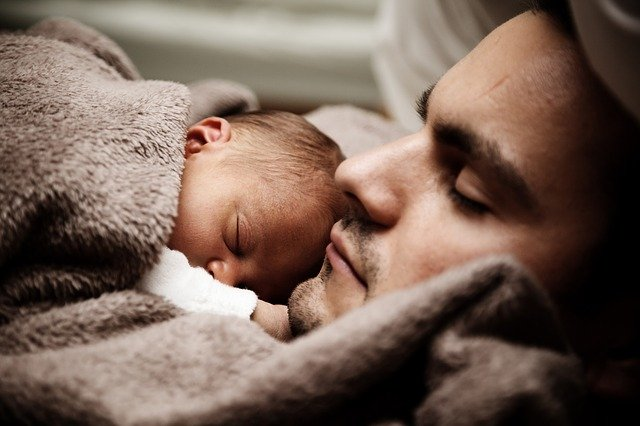## 16.3 Relative frequency approachWhat is the probability that a new baby will be a boy? The sample space could be listed as: boy and non-boy. The classical approach could be used, since the sample space has two elements: $$1\div2 = 0.5$$. This approach is fine if boys and non-boys are equally likely to be born. But are they?

In Australia in 2015, 305 377 live births occurred, with 157 088 male births and 148 289 non-male births. Then, the proportion of boys born in 2015 is

$\frac{157\,088}{305\,377} = 0.514,$ or about 51.4%. An estimate of the probability that the next birth will be a boy is is about 0.514 (or 51.4%). This is the relative frequency approach to calculating probabilities: based on past proportions.

The probability that the next birth will be a boy is approximately 0.514, but the next birth will either be a boy, or will be a not-boy… and we don’t know which will occur.

Definition 16.5 (Relative frequency approach to probability) In the relative frequency approach to probability, the probability of an event is (approximately) the number of times the outcomes of interest has appeared in the past, divided by the number of ‘attempts’ in the past.

Example 16.8 (Relative frequency probability) Based on this information, the odds that a new baby will be a boy is approximately $$0.514\div (1-0.514) = 1.058$$.

According to the ABS:

The sex ratio for all births registered in Australia generally fluctuates around 105.5 male births per 100 female births.

This is close to the odds of 1.058 found above.

Think 16.1 (Probability) The data in Table 16.1 concern students enrolling in a library introductory session in O-Week. (SSE is the School of Science and Engineering; SHSS is the School of Health and Sport Science.)

Find the probability that a randomly chosen student will be:

1. An SSE student.
2. An SHSS student aged Over 30.
3. Over 30, if we already know the student is from SHSS.
1: $$159/255 = 62.4$$%; 2: $$40/255=15.7$$%; 3: $$40/96 = 41.7$$%.
TABLE 16.1: Attendance at a library O-Week session
30 and under Over 30 Total
SHSS 56 40 96
SSE 68 91 159
Total 124 131 255